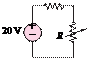### Create an Account

Already have account?

### Forgot Your Password ?

Home / Questions / For the circuit shown in Figure P235 determine the power absorbed by the variable resistor...

# For the circuit shown in Figure P235 determine the power absorbed by the variable resistor R ranging from0 to 20 Q Plot the power absorption as a function of R 5 Ù

For the circuit shown in Figure P2.35, determine the power absorbed by the variable resistor R, ranging from0 to 20 Q. Plot the power absorption as a function of R.

5 ÙJun 19 2020 View more View Less

#### Answer (Solved)Subscribe To Get Solution